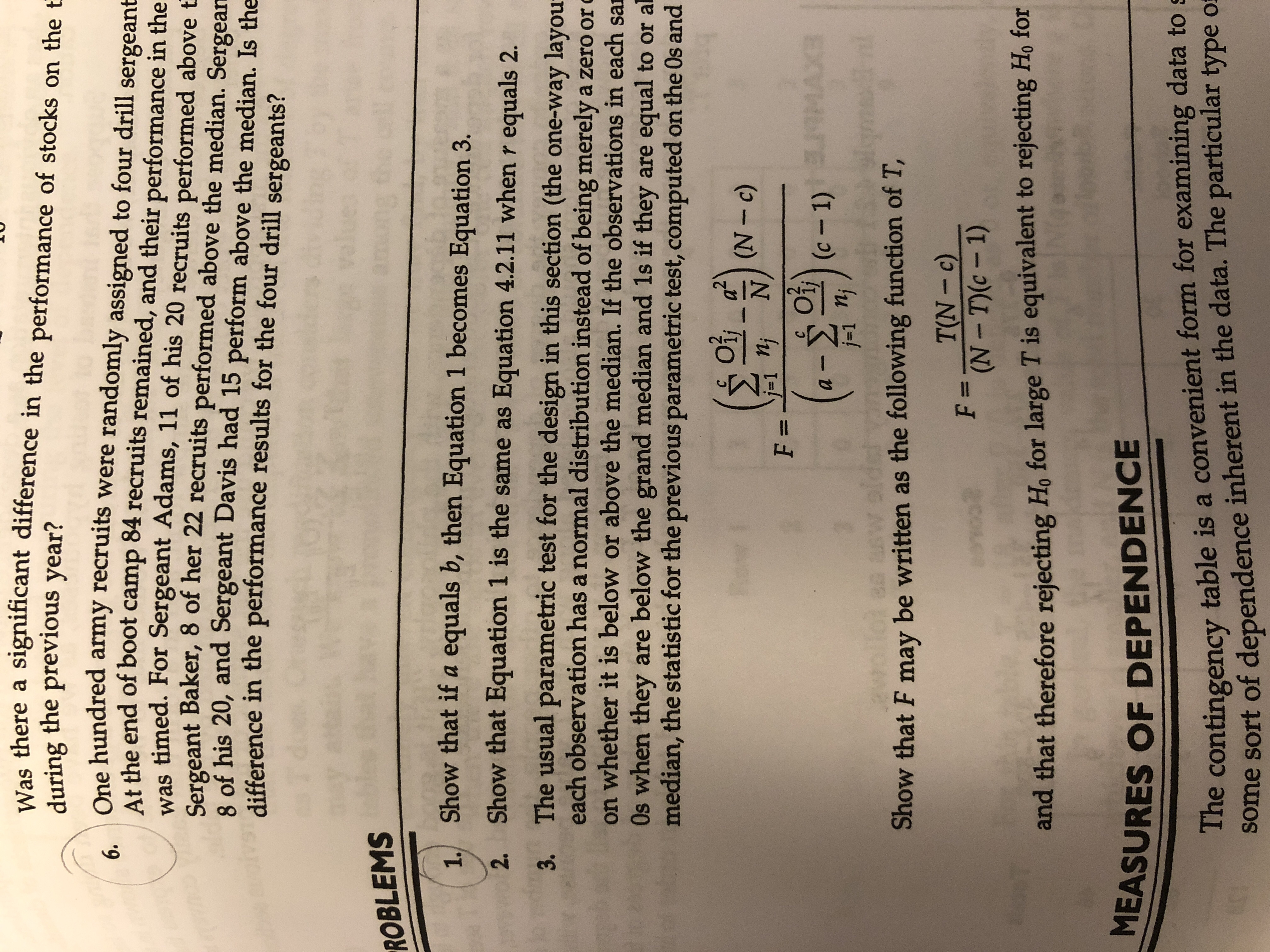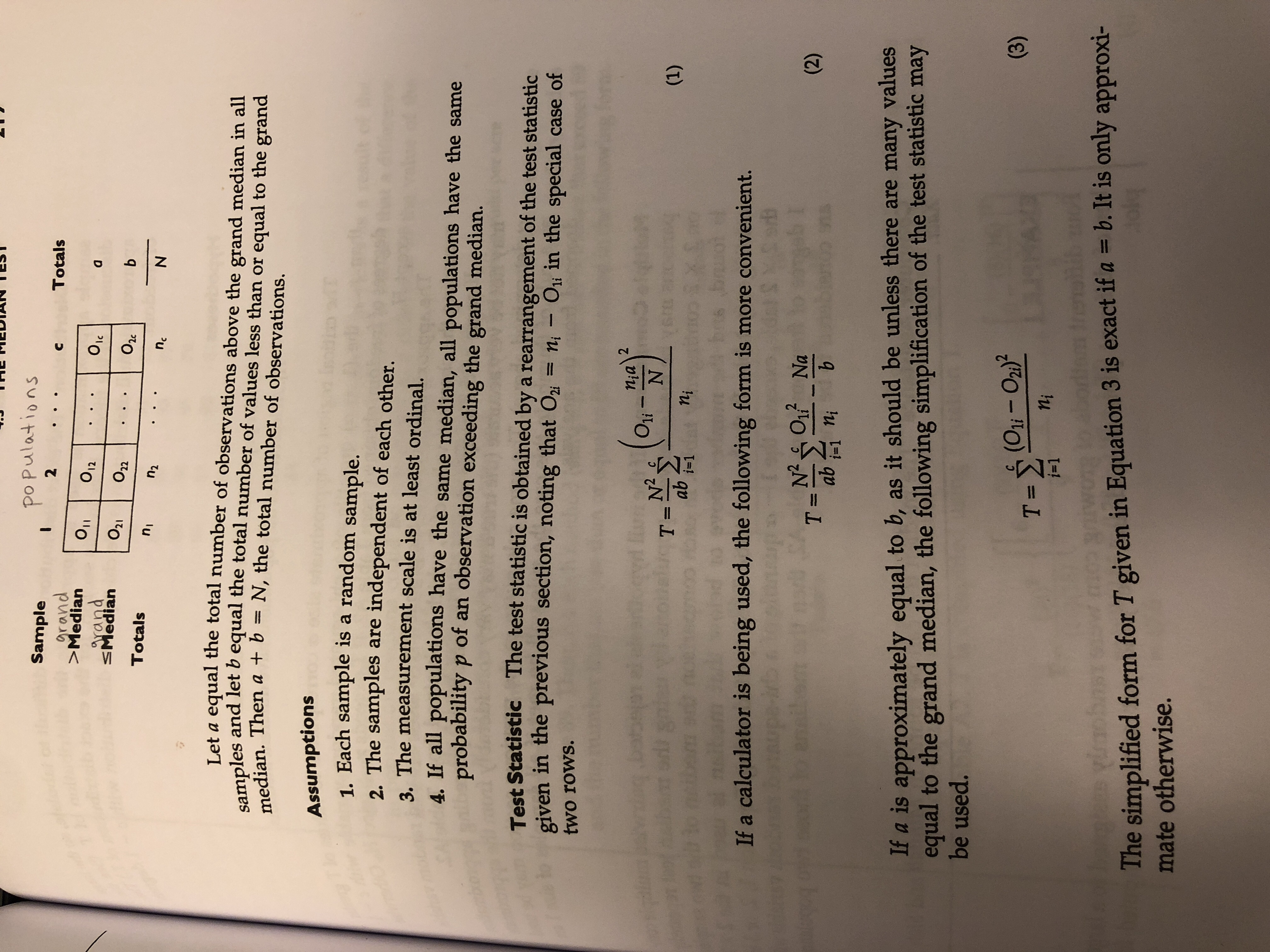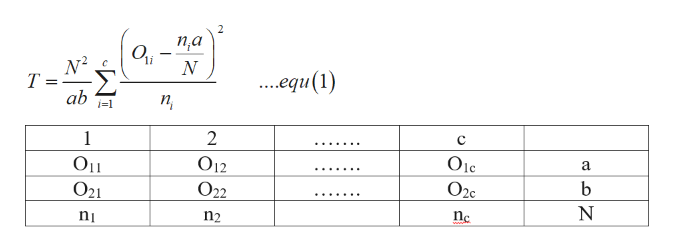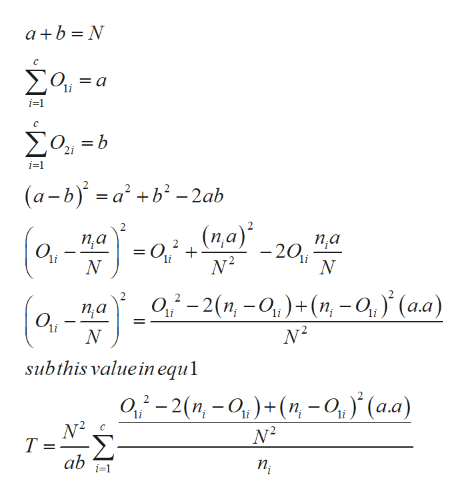# Was there a significant difference in the performance of stocks on the tduring the previous year?Уear?6.One hundred army recruits were randomly assigned to four drill sergeantAt the end of boot camp 84 recruits remained, and their performance in theras timed. For Sergeant Adams, 11 of his 20 recruits performed above tSergeant Baker, 8 of her 22 recruits performed above the median. Sergeamn8 of his 20, and Sergeant Davis had 15 perform above the median. Is thedifference in the performance results for the four drill sergeants?ROBLEMS1Show that if a equals b, then Equation 1 becomes Equatiorn 3.2.Show that Equation 1 is the same as Equation 4.2.11 when r equals 2.The usual parametric test for the design in this section (the one-way layoueach observation has a normal distribution instead of being merely a zero oron whether it is below or above the median. If the observations in each sa3.Os when they are below the grand median and 1s if they are equal to or almedian, the statistic for the previous parametric test, computed on the 0s and2(N- c)wn'jj-1 niF =9MAXG(c - 1)j-1 njShow that F may be written as the following function of T,T(N-c)F =(N-T(c- 1)and that therefore rejecting Ho for large T is equivalent to rejecting Ho forMEASURES OF DEPENDENCEThe contingency table is a convenient form for examining data to s609some sort of dependence inherent in the data. The particular type of Po Pulations2SampleTotalsgrand>MedianОтO12arandSMedianO21O22O2cbTotalsn2NncLet a equal the total number of observations above the grand median in allsamples and let b equal the total number of values less than or equal to the grandmedian. Then a b = N, the total number of observations.Assumptions1. Each sample is a random sample.2. The samples are independent of each other.3, The measurement scale is at least ordinal.4, If all populations have the same median, all populations have the sameprobability p of an observation exceeding the grand median.Test Statistic The test statistic is obtained by a rearrangement of the test statisticgiven in the previous section, noting that O ni- Oi in the special case oftwo rows.niaОliN2T =ab(1)i-1booIf a calculator is being used, the following form is more convenient.bO1 Na(2)T =abbniIf a is approximately equal to b, as it should be unless there are many valuesequal to the grand median, the following simplification of the test statistic maybe used.(3)T= (O-O2)2nii 1The simplified form for T given in Equation 3 is exact if a b. It is only approxi-mate otherwise.

Question
34 views

Question 1, equation 1 and equation 3 are in the second image.help_outlineImage TranscriptioncloseWas there a significant difference in the performance of stocks on the t during the previous year? Уear? 6. One hundred army recruits were randomly assigned to four drill sergeant At the end of boot camp 84 recruits remained, and their performance in the ras timed. For Sergeant Adams, 11 of his 20 recruits performed above t Sergeant Baker, 8 of her 22 recruits performed above the median. Sergeamn 8 of his 20, and Sergeant Davis had 15 perform above the median. Is the difference in the performance results for the four drill sergeants? ROBLEMS 1 Show that if a equals b, then Equation 1 becomes Equatiorn 3. 2. Show that Equation 1 is the same as Equation 4.2.11 when r equals 2. The usual parametric test for the design in this section (the one-way layou each observation has a normal distribution instead of being merely a zero or on whether it is below or above the median. If the observations in each sa 3. Os when they are below the grand median and 1s if they are equal to or al median, the statistic for the previous parametric test, computed on the 0s and 2 (N- c) w n'j j-1 ni F = 9MAXG (c - 1) j-1 nj Show that F may be written as the following function of T, T(N-c) F = (N-T(c- 1) and that therefore rejecting Ho for large T is equivalent to rejecting Ho for MEASURES OF DEPENDENCE The contingency table is a convenient form for examining data to s 609 some sort of dependence inherent in the data. The particular type of fullscreenhelp_outlineImage TranscriptionclosePo Pulations 2 Sample Totals grand >Median От O12 arand SMedian O21 O22 O2c b Totals n2 N nc Let a equal the total number of observations above the grand median in all samples and let b equal the total number of values less than or equal to the grand median. Then a b = N, the total number of observations. Assumptions 1. Each sample is a random sample. 2. The samples are independent of each other. 3, The measurement scale is at least ordinal. 4, If all populations have the same median, all populations have the same probability p of an observation exceeding the grand median. Test Statistic The test statistic is obtained by a rearrangement of the test statistic given in the previous section, noting that O ni- Oi in the special case of two rows. nia Оli N2 T = ab (1) i-1 boo If a calculator is being used, the following form is more convenient. b O1 Na (2) T = ab b ni If a is approximately equal to b, as it should be unless there are many values equal to the grand median, the following simplification of the test statistic may be used. (3) T= (O-O2)2 ni i 1 The simplified form for T given in Equation 3 is exact if a b. It is only approxi- mate otherwise. fullscreen
check_circle

Step 1

To prove equation 1 is equal to equation 2.

If a=b in the following equation.help_outlineImage Transcriptionclose2 п,а N2 ..equ(1) т ab i-1 п 2 1 Olc а O12 Оп b O2c O22 O21 nc n2 fullscreen
Step 2

From the table, we understood that...help_outlineImage Transcriptioncloseab N Σο- i-1 C Σο,-b 2i i=1 (a-b)ab2ab (n,a) па па -20i О N 0,-2(n, -0)+(n, -0,y (a.a) (0-0 п,а li li li subthis value in equl 0-2(n,-O)+(n, - 0, (a.a) Т- ab п, i-1 fullscreen

### Want to see the full answer?

See Solution

#### Want to see this answer and more?

Solutions are written by subject experts who are available 24/7. Questions are typically answered within 1 hour.*

See Solution
*Response times may vary by subject and question.
Tagged in

### Statistics